# Stochastic Gradient Descent: An intuitive proof

In this article, we explain why stochastic gradient descent works. Our proof technique uses techniques from ordinary differential equation techniques, but no previous background on the topic is required.

This article serves as a friendly introduction to convergence proofs using Lyapunov functions before diving into the work from our research group:

# Introduction

From a mathematical perspective, a neural network is just a parameterized function. To train a neural network is simply to minimize a function:

We use the function f to abstract away the choice of the loss function. For example, we may want to minimize the mean-squared error of a fully-connected neural network with weights represented by w using input-output pairs (x,y):

We use this notation because our analysis will not depend on the choice of the loss function, neural network model or dataset.

# The Origin of Stochastic Gradient Descent

Feel free to skip this section if you are familiar with the intuition and formulation of the SGD algorithm.

Stochastic Gradient Descent (SGD) uses the traditional gradient descent step, but with stochastic gradients. Let’s get into what each idea means separately before we combine them.

Intuitively, a step of gradient descent (GD) is like going down a hill by walking in the direction that points down. However, the direction of “down” does not point directly at the minimum. Then, we would have no need for an algorithmic way to solve the problem. Instead, the “down” direction guarantees we only will descend after a small step, not that we will reach the bottom of the hill. In fact, if we take a step that is too big we might end up going up.

Another thing to consider is that gradient descent only finds local minima. For example, in our three-hill example above, the minimum that the gradient points to varies with location.

The formula for the gradient descent update at a given iteration i is:

The parameters (or weights) are updated using the previous value. This means we must choose an initial value, w₀. The initial value, as expected, will impact the minimum that is found. A stopping criterion is needed as well. A common one is to set a threshold on the value ∇f(wᵢ), that is, to stop when ∇f(wᵢ) is very small and the value of w is changing very little.

The value hᵢ is called the time-step — or in deep learning, the learning rate. In neural networks, this value is usually experimented with until a suitable sequence of values is found. If the learning rate is too large we may never converge to a solution, and if it is too small it may converge too slowly. Later, we will discuss how to choose it.

Stochastic gradients are inexact gradients, that is, different but approximately the same as the true gradient, ∇ f. Although a stochastic gradient could be anything, in training neural networks, the one used is called a mini-batch gradient. The mini-batch gradient is the gradient computed over some of the training examples, instead of using all the training dataset.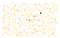The mini-batch gradient (pink triangles) points in the same approximate direction as the true gradient (orange circles). The mini-batch gradient is more efficient since it only uses a subset of points to be computed.

Stochastic gradients are used because of their computational and memory efficiency. Settings such as online learning may also prevent us from accessing the whole dataset.

Now, we take the formula from the gradient descent step and introduce a mini-batch gradient, to get a very similar expression:

SGD is implemented in all the main deep learning frameworks such as PyTorch and TensorFlow. It is the most widely used algorithm for training neural networks.

# Mathematical Notion of Convergence

A natural thing to do is get some math to back up the nice behaviour seen in practice when using SGD. For that, one must come up with a convergence proof. We say an algorithm converges if we are able to find a minimizer: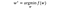The function f may have a minimum, but no minimizer. Take for example f(x) = 1/|x|.

Practically speaking, we want to show that as we iterate through the algorithm (i→∞), the value of the iterates approaches that of the minimum:Alternatively, one can say that for i large, ||wᵢ-w*|| is small.

Given an algorithm, it is usually easier to provide a bound on the difference ||wᵢ — w*||. For example, the following is enough to prove convergence:

Since a and b are constants, we say the above examples converges O(1/i). The above-normed difference is not the only possible quantity to prove convergence to a solution. We will see this in more detail later on.

Next, we bridge optimization and ordinary differential equations (ODEs) and explain Lyapunov functions.

# Optimization and Ordinary Differential Equations

The concept of Lyapunov functions — which we explain later — comes from the field of ODEs. There is a strong connection between ODEs and algorithms like gradient descent, but it might not be immediately obvious.

First, rearrange the formula for gradient descent to get: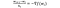We only moved some terms from the right to the left.

The term on the left is the finite difference approximation (FD) of the derivative of a continuous function ​w. Hence, we can say the above expression is a discretization of the ODE:

Now, in this framework, we are thinking of w as a continuous function, that evaluated at time tᵢ yields iterate wᵢ from gradient descent. We do not know the exact values of each tᵢ, but we do know from the FD that they are spaced by hᵢ. Even though imperfect, we can now transform mathematical concepts from optimization into ODEs and vice-versa.

Let’s start with the notion of a minimum of a function. Earlier, we referred to the minimizer of f as w*. Since w* is a local minimum of f, it has zero gradient,∇ f (w*) = 0. For a given ODE, u is an equilibrium point if it is constant over time — or, in the case of the above ODE,∇ f (u) = 0. Hence, w* is an equilibrium point.

However, being an equilibrium point for the ODE does not imply being a minimizer of f. Take for example the maximum of f, where we also have ∇ f (w*) = 0. Think back to the gradient descent algorithm. Since we are taking steps in the “down” direction, no matter how close we start to the maximum we will walk away from it. There are also points with zero gradient that are neither maximum nor minimum — saddle points.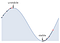The maximum — unstable — and the minimum — stable — are represented in black. We see two possible conditions — in pink — diverging and converging, respectively. While both are equilibrium points for the ODE, only one is a minimizer.

Considering the ODE, an equilibrium point u is said to be stable if starting with w(t)=u close enough to w* leads to a diminishing difference ​​|w(t) -w*| as time goes to infinity. ​We see in the above picture how this is true for minima, but not for maxima. It will also not be necessarily true for other stationary points. This means we can think of the minimum w* as a stable equilibrium point for the ODE. It means that if we solve the ODE and find a stable equilibrium point, then we have found a minimum of f. We will study those using Lyapunov functions, which we explain next.

# Lyapunov Functions

We start with an example, but we will refer in parenthesis to the property of the formal definition. We recommend ignoring this information before reading the mathematical definition.

Lyapunov functions are used to prove the stability of equilibrium points in ODEs. Think of a Lyapunov function as representing a physical system’s energy, for example, the energy of a ball at different positions on a hill. Note that energy is computed using continuous functions (Property 1).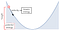The energy of the ball at a certain position is the sum of the potential energy (larger for greater heights) and the kinetic energy (increases with velocity).

In a real-world setting — where friction will exist — if the ball is released at the top of the hill, it will eventually stop at the bottom, even if it oscillates for some time.

In this case, the bottom of the hill is a stable equilibrium point for some ODE that can be derived from the laws of motion. It is natural to think of this equilibrium point ​as a point of no energy (Property 2). Also, the ball will stop at the bottom of the hill and nowhere else (Property 3).

Since friction is acting on the ball causing it to lose energy, and no other force is acting on the system, we may also say the system is not increasing in energy (Property 4). We see in the next image that while height goes up and down the energy always decreases.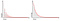These plots show how height and energy vary for an example like the one above.

Now that we have an intuition for each property, we proceed to the mathematical definition of a Lyapunov function, following this note. The standard definition usually assumes w*=0​ , we change it for clarity — but our definition remains equivalent. Here, wᵢ can be any sequence but think of it as the gradient descent sequence.

Definition:

A Lyapunov function is a continuous function ​E: ℝⁿ →ℝ such that:

Property 1. E​ is continuous;

Property 2. E(w)=0 if and only if ​w=w*;

Property 3. E(w)>0​​ if and only if ​w≠ w*;

Property 4. E(w₊₁)≤ E(w)​ for all i∈ℕ​.

Now that we understand the intuition for Lyapunov functions, how do we find one given the problem context? Well, the best part of Lyapunov functions is that it doesn’t matter if the energy has any physical meaning. If we find a function that satisfies Properties 1–4, it does not matter if it does not have an interpretation that translates to the real world. On one hand, in physics, Lyapunov functions do not have to be derived from physical laws. On the other hand, Lyapunov functions can be used in problems from fields where energy is not a well-defined concept, such as economics.

Unfortunately, to find a Lyapunov function we must use a trial-and-error approach. With a mix of intuition and experience, we can define several candidates and then check if the above properties hold. Properties 1–3 are usually quite straightforward, and the bulk of the proof time is spent on Property 4 —we will see later this is the property from which we will derive a convergence rate.

Assume we found a Lyapunov function for whatever problem we have at hand. We may know an equilibrium point exists as well — otherwise, the problem is not well-defined, and we should not be using gradient descent. Also, f should be a reasonable function — if not, mathematically, we can’t really guarantee any much. Then, we can prove that the existing equilibrium point is indeed stable, that is,

or — formally —

Theorem:

Assume the following:

Condition 1.w* is an equilibrium point for w₊₁ = G(h,w)​, that is, ​w* = G(h, w*) for all choices of hᵢ;

Condition 2. G​ is locally Lipchitz on ​ℝⁿ;

Condition 3. E​ be a Lyapunov function for ​w*;

Then, w*​ is a stable equilibrium point.

In the case of gradient descent G(hᵢ, wᵢ) = wᵢ-hᵢ∇ f(wᵢ). Hence, the first condition translates to w*=w*-hᵢ∇ f(w*), which equivalent to saying the gradient is zero at w*. Recall that if the gradient is zero, then w* is a stationary point of f (minimum, maximum or saddle point). So we may update the above conditions to reflect the gradient descent case:

Condition 1 for GD. w* is a stationary point of f;

Condition 2 for GD. ∇ f is locally Lipshitz on ℝⁿ;

We have purposely avoided defining what it means for G to be locally Lipshitz — we only discuss this later, along with additional assumptions we make on f.

## Convergence for Lyapunov Function

From the start, we are interested in the idea of convergence. However, how does this relate to Lyapunov functions? Recall Property 2, E(wᵢ)=0​ if and only if ​wᵢ=w*, then we may update our convergence definition to:

As before, we can prove instead something like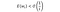Since 1/i→0 when i→∞, the above expression implies convergence of the energy to zero.

# Assumptions

As mentioned before, we need to assume the function f is “nice enough” in order to prove convergence and respective rates. The assumptions that follow might feel too restrictive, but they may hold locally, that is, close to the minimum they are likely to be true.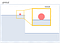In this example, the ball is not going anywhere because f is too flat. While globally it won’t satisfy our assumptions, locally (in yellow ) it does.

Our first assumption is strong convexity. We say a function f: ℝⁿ→ℝ is μ-convex when, for all x,yℝ:

This means, that for any point of f, there is a quadratic function that bounds the growth of the function. It is stronger than convexity, which only requires a linear bound. Given the graph of a function, we can think of convexity as can we draw a line under the graph for every point, and strong convexity as can we draw a quadratic μx² under each point of the graph.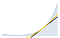We show a (black) line and a (yellow) quadratic are always below the example function, proving convexity and strong convexity, respectively.

Our second assumption is strong smoothness and can be proven to be the complementary definition of μ-smoothness. We say a function f: ℝⁿ→ℝ is L-smooth if the gradient ∇ f is L-Lipshitz. Recall that Condition 2 for GDintroduced in the context of the Theorem for Lyapunov functions — states the same. However, we have still not explained what is a Lipshitz function.

We say g: ℝⁿ→ℝ is L-Lipshitz if, for all x,yℝ,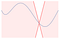Graphically, this constraint means that at each point of the function we can draw two intersecting lines, between which the function evolves. We limit linearly how much a function can change.

Curiously, ∇ f being L-Lipshitz as defined above implies an expression very similar to that for μ-convexity:

The intuition is very similar to the one for strong convexity, except now we need to be able to draw a quadratic Lx² above the function f at all points.

Actually, for L-smoothness we will be using another expression, that will be more suitable for our proof later on, but represents the exact same behaviour:

Before we continue, here are several different example functions that satisfy and don’t satisfy the assumptions we described in this section.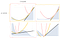The pink quadratic represents strong smoothness and must be above the graph. The yellow quadratic represents strong smoothness and must be below the graph. The black line represents convexity and must also be below.

Download or make your own copy of this Mathematica notebook if you want to experiment with parameters μ and L and graph locations:

We are now ready to move on to the convergence proofs. We will look at the simple cases for GD and SGD. In both cases, we will be using the tools from before. First, we define an energy function. Then, we show this energy is a Lyapunov function. Finally, we bound the energy to get a convergence rate.

We define energy as:

We can easily check Properties 1–3 to be a Lyapunov function hold. Property 1 holds because the energy function is a composition of continuous functions. Property 2 and 3 hold by definition of the norm. As we mentioned before, we need only focus on proving Property 4.

Property 4. E(w₊₁)≤ E(w)​ for all i∈ℕ​.

Proof: We prove the difference E(wᵢ₊₁)-E(wᵢ) is negative. First, we rewrite this difference using an algebra trick:

Next, we replace the difference wᵢ₊₁-wᵢ by the gradient descent step:

We now bound the new expression using strong convexity and smoothness: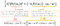Zoom in to see details on how we obtain the final expression.

We simplify the last expression and further bound to get: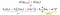The term on the left is always negative because of hᵢ≤1/L and f(w*)≤f(wᵢ).

Which gives us the final expression:

Since the learning rate, the μ constant and the energy E are always positive, we can say that the difference is always negative, proving Property 4.

End of Proof.

Now that we have proven E is indeed a Lyapunov function, we can use the Theorem to say that gradient descent will converge to w*. Moreover, the convergence rate follows easily from the final expression in the proof: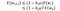The smallest is r=1-μhᵢ, the faster it converges. So we choose hᵢ=1/L for all i.

We define the energy the same way as for gradient descent:

As before, we focus on proving Property 4.

Property 4. E(w₊₁)≤ E(w)​ for all i∈ℕ​.

Proof: At the beginning of the proof, we follow the same steps as for GD. First, we rewrite the difference E(wᵢ₊₁)-E(wᵢ) using the same algebra trick. Then, we replace the difference wᵢ₊₁-wᵢ by the stochastic gradient descent step:

Our new expression includes stochastic gradients. However, our convexity and smoothness assumptions from before are for exact gradients. Before we proceed we notice some convenient properties of the mini-batch gradient. First, the average overall possible mini-batches is the exact gradient. Second, if the exact gradient, which is represented by a finite sum, is bounded, then so are mini-batch gradients, because they are partial sums. Hence, a natural assumption on a general stochastic gradient is:

Next, to remove stochastic gradients from our expression, we will bound the expectation of the difference, 𝔼[E(wᵢ₊₁)-E(wᵢ)]:

We may now bound the above expression using strong convexity and a gradient bound. We use strong convexity twice — one of them in a similar way to GD. For the gradient bound, we assume the gradient norm is bounded, a natural assumption for a discrete algorithm.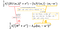The derivation of the expression in the first yellow box for μ-convexity is in the proof for GD.

We may simplify the last expression to get the overall expression:

which gives us:

Unfortunately, we cannot say straight away the difference is always negative since the difference is bounded by a sum of positive and a negative expression. Hence, we are going to get a convergence rate expression first from the above expression and use that inequality to prove Property 4.

The convergence rate of E (not yet proven to be a Lyapunov function) is: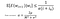We can see the rate improves with the constant μ, which means that the stronger the convexity, the faster the convergence. Conversely, the rate worsens with both constants M and σ.

Proof of Convergence Rate:

Now, that we have the convergence rate and the corresponding choice of the learning rate, we prove the difference is negative:

End of Proof.

We have proven E is indeed a Lyapunov function. Hence, we can use the Theorem to say that stochastic gradient descent will converge to w*. Moreover, we found the convergence rate during our proof. Therefore, SGD converges with rate O(1/i).

# Conclusion

In this article, we proved convergence for both gradient descent and stochastic gradient descent and provide rate constants. Not only that, but we explained the connection between the optimization of neural networks and solving and ordinary differential equations in discrete-time. That connection allowed us to introduced powerful tools such as Lyapunov functions in the context of neural networks.

This approach has been used in recent articles to prove different convergence results for methods such as Heavy-ball, Nesterov, and Polyak.

Written by

Written by

## Mariana Oliveira Prazeres

#### Trained mathematician, accidental deep learning researcher. Student at McGill University and part-time researcher at Huawei.